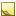﻿Number data typeNumber data type

The data type Number represents a numeric value, without units.

Unless specified, number data type values use a 64-bit floating point format (double). Fields that require higher precision like those that store financial results or monetary data use a 128-bit format (decimal).Usage

In the [Output] section, there are no units to covert:

```[Output]
MandatedSpeedLimit = input.SpeedLimit```Field length

The field length for the Number data type varies based on the format of the field value.

Value typeApproximate RangePrecision
double (default)(±5.0 x 10-324 to ±1.7) × 1030815-16 digits
decimal(-7.9 x 1028 to 7.9 x 1028)/(1000 to 28)28-29 digitsNoteJavaScript conversion

When you use the optional [Script] section to insert your own JavaScript functions, note that Number maps to JavaScript Number, with JavaScript native number assignment and full use of number operators.

Creation
JavaScript
`var myNumber = 7;`
Properties

These are the native JavaScript properties for Number objects:

• constructor — Returns the function that created the Number object's prototype

• MAX_VALUE — Returns the largest number possible in JavaScript

• MIN_VALUE — Returns the smallest number possible in JavaScript

• NEGATIVE_INFINITY — Represents negative infinity (returned on overflow)

• NaN — Represents a "Not-a-Number" value

• POSITIVE_INFINITY — Represents infinity (returned on overflow)

• prototype — Allows you to add properties and methods to an object

Methods

These are the native JavaScript methods for Number objects:

• toExponential(x) — Converts a number into an exponential notation

• toFixed(x) — Formats a number with x numbers of digits after the decimal point

• toPrecision(x) — Formats a number to x length

• toString() — Converts a Number object to a string

• valueOf() — Returns the primitive value of a Number object